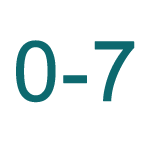Report a Problem
Suggestions

#Octal Number Converter

Last updated: Saturday, April 29, 2023

Octal number is a base-8 number system that uses eight digits, ranging from 0 to 7. This means that in octal, there are only eight distinct symbols used to represent numbers, which makes it a simpler system than the more commonly used base-10 decimal system. Octal numbers are often used in computer programming because they can be easily converted to binary, which is the language that computers use to store and manipulate data.

Converting octal numbers to other number systems, such as base-10 decimal, binary, or hexadecimal, is a common task in computer programming. In order to convert an octal number to decimal, you must first understand the place value system used in octal. Each digit in an octal number represents a power of 8, with the rightmost digit representing 8^0 (which equals 1), the next digit to the left representing 8^1 (which equals 8), and so on. To convert an octal number to decimal, you simply multiply each digit by its corresponding power of 8 and add up the results.

Converting octal numbers to binary or hexadecimal is also relatively simple, because each octal digit can be easily represented as a sequence of binary digits or a single hexadecimal digit. For example, the octal number 642 can be converted to binary by simply replacing each octal digit with its corresponding 3-bit binary sequence, resulting in the binary number 110 100 010. Similarly, the octal number 642 can be converted to hexadecimal by replacing each octal digit with its corresponding hexadecimal symbol, resulting in the hexadecimal number 0x34A.

Please enter a whole number (Base 10 Numeral)
Please enter a hex number (Base 16 Numberal)
Please enter a binary number (Base 2 Numeral)